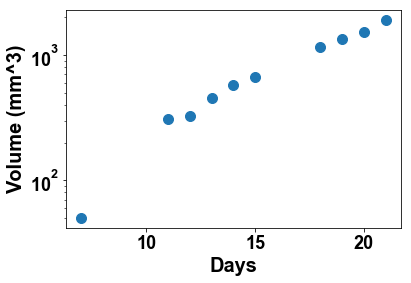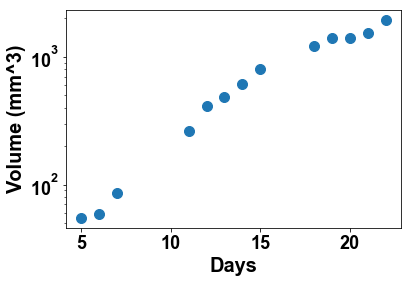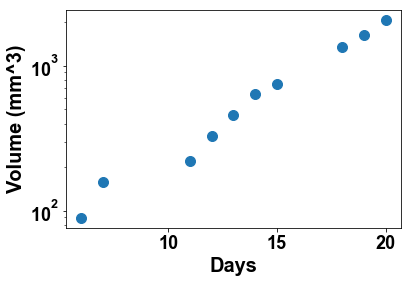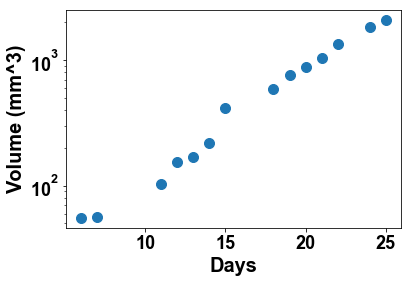# Tumor growth modeling¶

This practical session is intended to explore tumor growth data and interpret it using mathematical models. It is divided into three parts (only the first two parts will be covered in this session):

1. Analysis of the data by basic plots
2. Fitting and comparing tumor growth models to the data in order to understand tumor growth laws
3. Using the model(s) to predict future tumor growth with only a limited number of initial data points

The data provided consists of measurements of tumor volumes from tumors implanted subcutaneously in the back of mice. The cells are from a murine lung cancer cell line (Lewis Lung Carcinoma). The volumes were computed from two one dimensional measures recorded using a caliper (the length $L$ and width $w$) and using the formula $V = \frac{1}{2}L\times w^2$. Volumes are given in mm$^3$ as a function of days following injection of the cells ($10^6$ cells $\simeq$ 1 mm$^3$ injected on day 0.

# 1. Data analysis¶

### Import modules¶

In [ ]:
% matplotlib inline

In :
import numpy as np
import pandas as pd
import matplotlib.pyplot as plt


Load the data file data_table.xlsx as a pandas Dataframe and display it

In :
df = pd.read_excel('data_table.xlsx')
df

Out:
1 2 3 4 5 6 7 8 9 10
5 NaN 54.886377 NaN NaN 68.809022 NaN NaN NaN NaN NaN
6 NaN 59.408036 89.231295 55.348590 59.853289 46.502387 94.727972 NaN 18.567329 26.880016
7 50.021795 85.662278 157.351502 56.175814 58.988502 55.155193 126.094176 NaN 73.293694 NaN
11 309.519493 261.572775 221.698580 103.330909 179.664759 251.222209 333.136619 201.165288 126.820889 144.151300
12 324.895878 412.265650 327.492254 155.320341 309.787647 341.175444 538.680724 316.382967 222.661158 193.401925
13 450.842120 488.450738 461.437963 167.958671 470.571322 438.335387 669.929621 338.240373 244.726914 281.171233
14 572.450814 618.854795 641.444986 219.378690 480.930314 859.952765 762.527617 411.958788 333.629836 294.886207
15 664.336606 798.997212 746.868414 412.378378 488.777983 854.727952 923.717646 586.667016 367.475268 391.884141
18 1151.693754 1218.721058 1359.458389 584.192211 1021.721046 1143.279505 NaN 991.881984 805.778850 744.954870
19 1338.383299 1415.471856 1626.874371 762.074018 1278.530775 1645.406820 NaN 1219.899900 1030.034281 990.331786
20 1522.807849 1410.149208 2063.912472 880.325721 1377.381845 1950.482691 NaN 1833.096551 1272.818884 1085.314905
21 1897.073737 1524.126579 NaN 1040.074430 1538.922047 NaN NaN 2131.605693 1555.359077 1331.189667
22 NaN 1935.415344 NaN 1335.136464 1958.560253 NaN NaN NaN 1671.148523 1641.333918
24 NaN NaN NaN 1850.447333 NaN NaN NaN NaN NaN 1992.067465
25 NaN NaN NaN 2079.445167 NaN NaN NaN NaN NaN NaN

Get the time vector. It is in days

In :
time = df.index
time

Out:
Int64Index([5, 6, 7, 11, 12, 13, 14, 15, 18, 19, 20, 21, 22, 24, 25], dtype='int64')

Plot the growth of the first three mice.

In :
# Mouse 1
plt.figure(1)
plt.plot(time, df, 'o')
plt.xlabel('Days')
plt.ylabel('Volume (mm^3)')

# Mouse 2
plt.figure(2)
plt.plot(time, df, 'o')
plt.xlabel('Days')
plt.ylabel('Volume (mm^3)')

# Mouse 3
plt.figure(3)
plt.plot(time, df, 'o')
plt.xlabel('Days')
plt.ylabel('Volume (mm^3)')

Out:
Text(0,0.5,'Volume (mm^3)')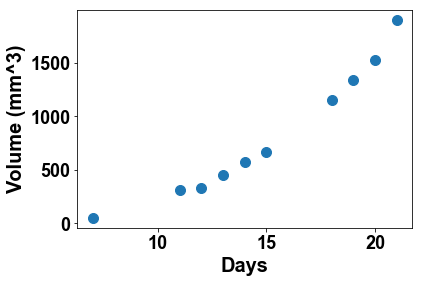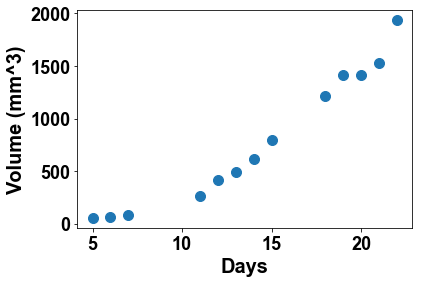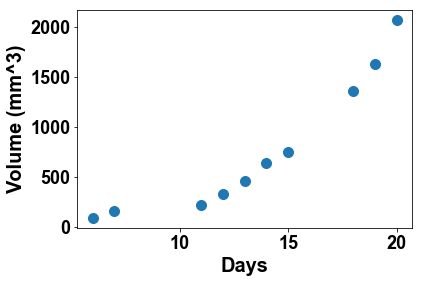Plot all tumor growth on the same panel

In :
for mouse in df.columns:
plt.plot(time, df[mouse], 'o')
plt.xlabel('Days')
plt.ylabel('Volume (mm^3)')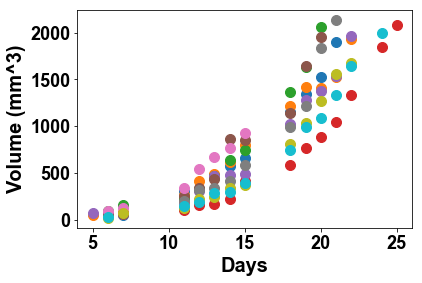Plot the average of the data with error bars as standard deviations

In :
# Generate columns with mean
df['mean'] = df.mean(axis=1)
# Generate columns with std
df['std'] = df.std(axis=1)
df[['mean']].plot(fmt='o', yerr=df['std'])
plt.legend(loc='upper left')
plt.xlabel('Days')
plt.ylabel('Volume (mm^3)')

Out:
Text(0,0.5,'Volume (mm^3)')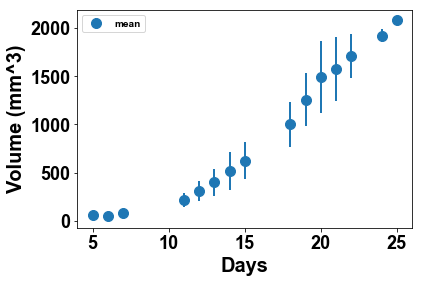From the individual plots, what tumor growth pattern/equation would you suggest? How could you simply graphically test it? What do you conclude?

In :
# Exponential growth
# Should be linear in log scale
# Mouse 1
plt.figure(1)
plt.semilogy(time, df, 'o')
plt.xlabel('Days')
plt.ylabel('Volume (mm^3)')

# Mouse 2
plt.figure(2)
plt.semilogy(time, df, 'o')
plt.xlabel('Days')
plt.ylabel('Volume (mm^3)')

# Mouse 3
plt.figure(3)
plt.semilogy(time, df, 'o')
plt.xlabel('Days')
plt.ylabel('Volume (mm^3)')

# Mouse 4
plt.figure(4)
plt.semilogy(time, df, 'o')
plt.xlabel('Days')
plt.ylabel('Volume (mm^3)')

Out:
Text(0,0.5,'Volume (mm^3)')
/Users/benzekry/anaconda3/lib/python3.6/site-packages/matplotlib/scale.py:111: RuntimeWarning: invalid value encountered in less_equal
out[a <= 0] = -1000